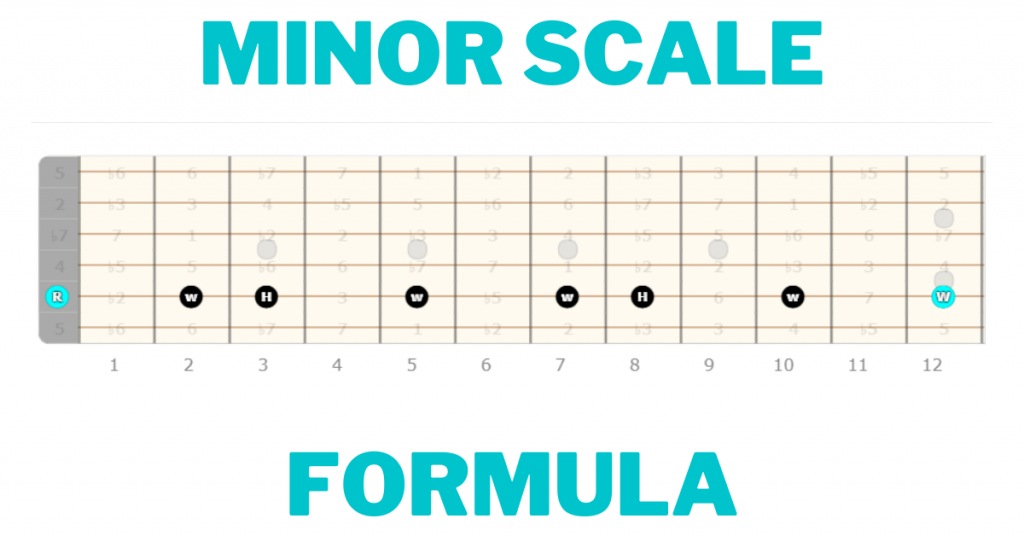# Minor Scale Formula Explained | Guitar LessonIn this lesson you’ll learn the minor scale formula, how to apply it to your guitar playing, and how it differs from the major scale!

Let’s get to it!

## What is the minor scale formula?

The minor scale formula is built from this formula of whole and half steps:

W-H-W-W-H-W-W

## Building the natural minor scale step-by-step

Now that you know your minor scale formula it’s time to pick a root note and apply it!

Let’s do this step-by-step.

1. Pick your root note. You’ll use the root note A, which will result in the A minor scale.
2. Use the open A string as your note for this example.
3. Starting from the root, move up a whole step (W), which gets you to the B note.
4. Continue to follow the formula of whole and half steps until you get back to the A note.
5. The end result will be the notes A-B-C-D-E-F-G-A, giving you every note in the A minor scale and ending on the octave.

## Major Vs. Minor Scale

To better understand the construction of the minor scale, let’s look at how it derives from and compares to the major scale.

You’ll use the C major scale for the example, because it has not sharps or flats.

### Major scale intervals

The major scale interval structure is 1-2-3-4-5-6-7.

Applying this to the root note C gives you the C major scale.

### Minor scale intervals

The minor scale interval structure is 1-2-b3-4-5-b6-b7.

Applying this to the root note C gives you the C minor scale.

### The difference

The difference between the major and minor scale boils down to the 3rd, 6th, and 7th intervals. All of these intervals are natural notes in the major scale, and they’re flat notes in the minor scale.

After you learn how to play the minor scale, you’ll learn about parallel and relative minor scales.

## Playing and applying the minor scale formula

These examples use the A minor scale. You can transpose them as needed.

### 3-notes-per-string minor shape

The 3 notes-per-string minor shape is one of seven shapes used to visualize the fretboard.

### Minor CAGED shape

The minor CAGED shape is derived from the open E minor chord, which is outlined in the diagram below.

### Minor scale octave shape

You can apply this minor octave shape to any root note.

Try it using the two octaves in the diagram, then practice moving it to different A notes across the fretboard.

## Minor scale formula terms

### Parallel minor

If a major scale and a minor scale have the same root note, they are called “parallel” scales.

For example, C minor is the parallel minor of C major since the C major scale has the same root note as the C minor scale.

This works both ways, so you could call C major the parallel major of C minor.

### Relative minor

A relative minor is the minor scale derived from the sixth degree of a parent major scale.

For example, the relative minor of the C major scale is the A minor scale.

To visualize this, here are the notes in the C major scale:

If you then build this scale from the 6th note, which is A, the result is this:

As you can see, the notes remain the same, but the interval structure changes. This results in the relative minor.

Whether or not the group of notes is major or minor is determined by the tonal center. The tonal center is simply the root note displayed by the harmony and context of the music.

## Minor scale formula conclusion

The minor scale can be made using the formula W-H-W-W-H-W-W, or by using it’s interval structure 1-2-b3-4-5-b6-b7.

Use the minor scale shapes as shown above to practice the scale and apply it to your own guitar playing.

Thanks for reading, and let me know if you have any questions in the comments section.